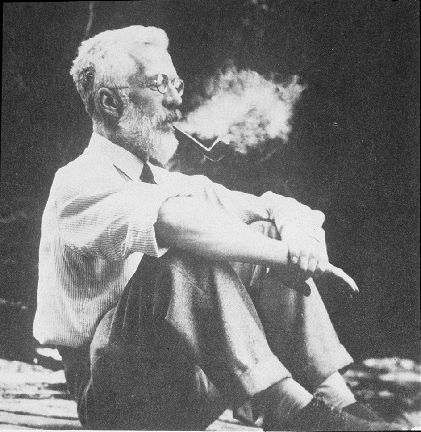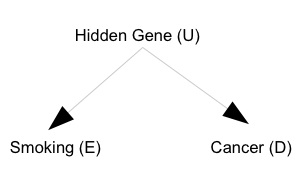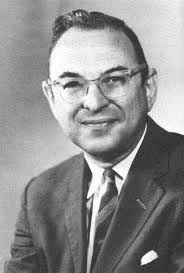# 一、Cornfield 条件或者 Cornfield 不等式（图注：R A Fisher）$$RR_{ED}= \frac{ P(D=1\mid E=1) } { P(D=1\mid E=0) } = 9.$$（图注：J Cornfield）

Cornfield 通过简单的数学证明，得到了如下的不等式，文献中也称为 Cornfield 不等式：

$$RR_{EU} \geq RR_{ED}.$$

… if cigarette smokers have 9 times the risk of nonsmokers for developing lung cancer, and this is not because cigarette smoke is a causal agent, but only because cigarette smokers produce hormone X, then the proportion of hormone-X producers among cigarette smokers must be at least 9 times greater than nonsmokers. If the relative prevalence of hormone-X-producers is considerably less than ninefold, then hormone-X cannot account for the magnitude of the apparent effect.

Cornfield 的这项简单研究，开始了流行病学和统计学中敏感性分析的研究；比如 Rubin 和 Rosenbaum 很多工作都是在 Cornfield 的启发下做出来的。简单地说，敏感性分析，就是在朝着 Yule-Simpson Paradox 的反方向进行的：复杂虽然总是存在，但是我们相信这个世界并不是疯狂的复杂。

# 二、技术细节

$$\begin{eqnarray} f_1 = P(U=1\mid E=1), &&f_0 = P(U=1\mid E=0),\\ RR_{EU} = \frac{ P(U=1\mid E=1) }{ P(U=1\mid E=0)} = \frac{f_1} {f_0}, && RR_{UD} = \frac{ P(D=1\mid U=1) }{ P(D=1\mid U=0) }. \end{eqnarray}$$

$$\begin{eqnarray} RR_{ED} &=& \frac{ P(D=1\mid E=1) } { P(D=1\mid E=0) }\\ &=& \frac{ \sum_{u=0,1}P(D=1, U=u\mid E=1) } {\sum_{u=0,1} P(D=1, U=u\mid E=0) }\\ &=& \frac{ \sum_{u=0,1}P(D=1\mid U=u) P(U=u\mid E=1) } {\sum_{u=0,1} P(D=1\mid U=u) P(U=u\mid E=0) }\\ &=& \frac{ P(D=1\mid U=1)P(U=1\mid E=1) + P(D=1\mid U=0)P(U=0\mid E=1) } { P(D=1\mid U=1)P(U=1\mid E=0) + P(D=1\mid U=0)P(U=0\mid E=0) }\\ &=& \frac{ RR_{UD} f_1 + (1-f_1)}{ RR_{UD} f_0 + (1-f_0)}. \end{eqnarray}$$

$$RR_{ED} \leq \lim_{RR_{UD}\rightarrow +\infty} \frac{ RR_{UD} f_1 + (1-f_1)} { RR_{UD} f_0 + (1-f_0) } = \frac{f_1}{f_0} = RR_{EU}.$$

# 三、文献注记

Cornfield 最早的论文发表于 1959 年；由于它的重要性，这篇文章又在 2009 年重印了一次（50 周年纪念）。于是参考文献有两篇，它们是一样的；不过后者多了很多名人的讨论。

• Cornfield J et al. Smoking and lung cancer: recent evidence and a discussion of some questions. JNCI 1959;22:173-203.
• Cornfield J et al. Smoking and lung cancer: recent evidence and a discussion of some questions. Int J Epidemiol 2009;38:1175-91.（本文邀请了 David R Cox 和 Joel B Greenhouse 等人讨论。）